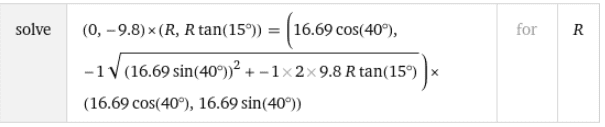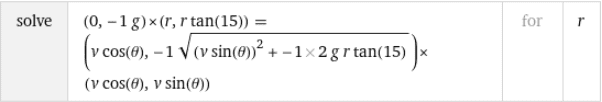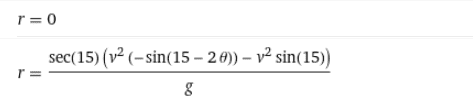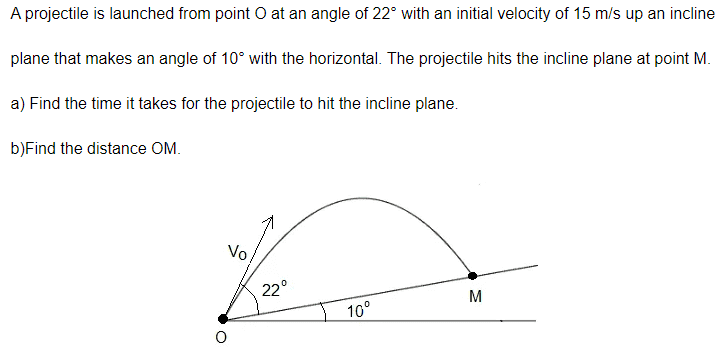# Projectile motion when kicking a ball that lands on an upward slope

gjh
Homework Statement:
A soccer player can kick the ball 28 m on level ground, with initial velocity at 40 degrees to the horizontal. At the same initial speed and angle to the horizontal, what horizontal distance can the player kick the ball on a 15 degree upward slope.
Relevant Equations:
trajectory equation: y = x*tan (theta)-[(g*x^2)/(2*v()^2*(cos(theta)^2) ... (1)
We know x = R =max range (28m) on level ground. Need to find v()^2. Subbing y=0 into (1) above, get v(0)^2 = (gR^2/)/(2*cos (theta)^2 * tan (theta). ... (2)
This didn't seem right, since this means v(0)^2 is a negative number ... maybe my orientation or algebra wrong?

Anyway, didn't see any other way to proceed, so I subbed value of v(0)^2 into (1) above, and let y = x*tan (theta 1) to represent the slope of the hill. Got substantial cancellation and final expression of x = R*[tan (theta (0) - tan (theta (1)]/tan (theta 0), where theta (0) is 40 degrees and theta (1) = 15 degrees. Then x = 19 m, which agrees with the numerical answer in the back of the book ... BUT don't understand how I can use an invalid interim result [square of a number is negative) to obtain a valid final result. Comments, please!

Homework Helper
Gold Member
Relevant Equations:: trajectory equation: y = x*tan (theta)-[(g*x^2)/(2*v()^2*(cos(theta)^2) ... (1)

We know x = R =max range (28m) on level ground. Need to find v()^2. Subbing y=0 into (1) above, get v(0)^2 = (gR^2/)/(2*cos (theta)^2 * tan (theta). ... (2)
This didn't seem right, since this means v(0)^2 is a negative number
There are only two ways that I can see how you got a negative number. Either your calculator is in radian mode or you substituted a negative value for g. I don't think you solved for ##v_0^2## correctly. You can see that your expression for ##v_0^2## does not have the correct dimensions.

Homework Helper
Gold Member
Start from$$0=x\tan\theta-\frac{gx^2}{2v_0^2cos^2\theta}$$Cancel what cancels.$$0=\sin\theta-\frac{gx}{2v_0^2cos\theta}$$Cross multiply and rearrange$$2v_0^2\sin\theta\cos\theta=gx$$etc. etc.

On edit:
I don't know what kind of magic you used to get the answer, but this is what I would do. When the projectile lands on the incline, its horizontal and vertical displacements are ##\Delta x## and ##\Delta y##. The trajectory equation becomes
$$\Delta y=\Delta x\tan\theta-\frac{g(\Delta x)^2}{2v_0^2cos^2\theta}$$ Divide by ##\Delta x##
$$\frac{\Delta y}{\Delta x}=\tan\theta-\frac{g(\Delta x)}{2v_0^2cos^2\theta}.$$
Assuming that you got ##v_0## correctly from the previous part, do you see how to proceed from here?

Last edited:
Homework Helper
Can use $$\vec{a} \times \vec{s} = \vec{v} \times \vec{u}$$ First:Then:Leads to a slightly 'ugly' quadratic but otherwise straightforward except that no-one save my learned colleagues contributing here will know what you're talking about!

Last edited:
•Delta2
Homework Helper
Gold Member
2022 Award
An alternative is not to calculate the initial velocity, but to derive an equation for the horizontal range on the slope (##r##) in terms of the full horizontal range (##R##):
$$r = R(1 - \frac{\tan \alpha}{\tan \theta})$$Where, in this case, ##R = 28m##, ##\theta = 40°## and ##\alpha = 15°##.

Last edited:
•neilparker62 and Delta2
Homework Helper
An alternative is not to calculate the initial velocity, but to derive an equation for the horizontal range on the slope (##r##) in terms of the full horizontal range (##R##):
$$r = R(1 - \frac{\tan \alpha}{\tan \theta})$$Where, in this case, ##R = 28m##, ##\theta = 40°## and ##\alpha = 15°##.
Ah yes - there's always a simpler way! Wolfram Alpha chugs through the literal equation:to obtain:which can be manipulated into the much simpler form shown by @PeroK above.

Last edited:
RBird
For a projectile going up an incline starting from (0,0), choose the x-axis as going up the incline. With ϕ as the angle of the incline from the horizontal, and θ as the angle of the initial velocity of the projectile as measured from the surface of the incline, then the impact occurs when y = $v_o$sin(θ)t + (1/2)[-g cos(ϕ)]$t^2$= 0, and x = $v_o$cos(θ)t + (1/2)[-g sin(ϕ)]$t^2$. Solve the y equation for t, and use that to find x = (2$v_o$/g)[sin(θ)cos(θ)/cos(ϕ) – sin(ϕ)si$n^2$(θ)/co$s^2$(ϕ)].
If the surface is horizontal, then ϕ =0 and x = (2$v_o$/g)sin(θ)cos(θ). This gives a positive ${v_o}^2$.

Homework Helper
Gold Member
2022 Award
For a projectile going up an incline starting from (0,0), choose the x-axis as going up the incline. With ϕ as the angle of the incline from the horizontal, and θ as the angle of the initial velocity of the projectile as measured from the surface of the incline, then the impact occurs when y = $v_o$sin(θ)t + (1/2)[-g cos(ϕ)]$t^2$= 0, and x = $v_o$cos(θ)t + (1/2)[-g sin(ϕ)]$t^2$. Solve the y equation for t, and use that to find x = (2$v_o$/g)[sin(θ)cos(θ)/cos(ϕ) – sin(ϕ)si$n^2$(θ)/co$s^2$(ϕ)].
If the surface is horizontal, then ϕ =0 and x = (2$v_o$/g)sin(θ)cos(θ). This gives a positive ${v_o}^2$.I think you are a dollar short on your Latex delimiters.

•berkeman
Homework Helper
Gold Member
For a projectile going up an incline starting from (0,0), choose the x-axis as going up the incline. With ϕ as the angle of the incline from the horizontal, and θ as the angle of the initial velocity of the projectile as measured from the surface of the incline, then the impact occurs when y = $v_o$sin(θ)t + (1/2)[-g cos(ϕ)]$t^2$= 0, and x = $v_o$cos(θ)t + (1/2)[-g sin(ϕ)]$t^2$. Solve the y equation for t, and use that to find x = (2$v_o$/g)[sin(θ)cos(θ)/cos(ϕ) – sin(ϕ)si$n^2$(θ)/co$s^2$(ϕ)].
If the surface is horizontal, then ϕ =0 and x = (2$v_o$/g)sin(θ)cos(θ). This gives a positive ${v_o}^2$.
There is no reason to solve for the time. As shown in post #3, the trak=jectory equation can be rewritten as $$\frac{\Delta y}{\Delta x}=\tan\theta-\frac{g(\Delta x)}{2v_0^2cos^2\theta}.$$Then one recognizes that ##\frac{\Delta y}{\Delta x} = \tan\varphi## and write $$\tan\varphi=\tan\theta-\frac{g(\Delta x)}{2v_0^2cos^2\theta}.$$if one has the value of ##v_0##, one can plug into find ##\Delta x##.

Alternatively, one can avoid pushing too many buttons on one's calculator and use the horizontal range when the projectile lands on level ground, $$R=\frac{2v_0^2\cos\theta\sin\theta}{g}=\frac{2v_0^2\cos^2\theta\sin\theta}{g\cos\theta}\implies \frac{1}{2v_0^2\cos^2\theta}=\frac{\tan\theta}{gR}$$Then the equation simplifies some more to$$\tan\varphi=\tan\theta-\frac{\Delta x}{R}\tan\theta$$which is @PeroK's expression in post #5.

•PeroK
Homework Helper
An alternative is not to calculate the initial velocity, but to derive an equation for the horizontal range on the slope (##r##) in terms of the full horizontal range (##R##):
$$r = R(1 - \frac{\tan \alpha}{\tan \theta})$$Where, in this case, ##R = 28m##, ##\theta = 40°## and ##\alpha = 15°##.
Geometric / graphic interpretation of this equation.

https://www.desmos.com/calculator/mfilokes7z

Last edited:
Homework Helper
Consider a general function of the form: $$f(x) = ax - bx^2=t(a-bx)$$ modeled after: $$\Delta y = \tan(\theta)x - \frac{gx^2}{2v^2}sec^2(\theta).$$ Plainly f(x) will have roots at ##x=0## and ##x=\frac{a}{b}##. Hence the "range" R (ie distance between the roots) will be ##\frac{a}{b}##. Let us now introduce a second function of the form ##g(x) = mx## modelling a slope of angle ##\alpha## such that ##\tan\alpha=m## and determine the point of intersection of f(x) and g(x): $$ax-bx^2=mx \implies x(a-m-bx)=0$$ $$\implies x=0\text{ or }x=\frac{a-m}{b}=R-\frac{m}{b}.$$ In the last expression above, we can remove ##R=\frac{a}{b}## as a common factor obtaining: $$x=R\left(1-\frac{m}{a}\right).$$ Finally writing ##m=\tan\alpha## and ##a=\tan\theta##, we obtain the expression presented by @PeroK :$$x(=r)=R\left(1-\frac{\tan\alpha}{\tan\theta}\right).$$

Homework Helper
I found a solution (per @PeroK 's expression above) and then went looking for a problem! Here it is:b) $$x = \frac{15^2\sin(64^{\circ})}{9,8}\times \left(1-\frac{\tan10^{\circ}}{\tan32^{\circ}}\right)\approx 14,81m$$ $$\implies OM=14.81 \sec10^{\circ}\approx15,04m$$ a) $$t=14,81 \div (15\cos32^{\circ}) \approx 1,16 s$$ This is Problem 2 from this set of problems.

Last edited:
Homework Helper
Gold Member
2022 Award
I found a solution (per @PeroK 's expression above) and then went looking for a problem! Here it is:

View attachment 303175
b) $$x = \frac{15^2\sin(64^{\circ})}{9,8}\times \left(1-\frac{\tan10^{\circ}}{\tan32^{\circ}}\right)\approx 14,81m$$ $$\implies OM=14.81 \sec10^{\circ}\approx15,04m$$ a) $$t=14,81 \div (15\cos32^{\circ}) \approx 1.16 s$$ This is Problem 2 from this set of problems.
Well, I never claimed it was original research!

•malawi_glenn
Homework Helper
Well, I never claimed it was original research!
Very useful all the same - for projectiles on inclined plane problems such as this. Another useful formula applicable when the projectile passes maximum height and then lands at a height different from launch height:
$$x=\frac{R}{2} \times \left (1+\left |\frac{v_{yf}}{v_{yi}}\right |\right)$$ eg Find time of flight for a rock hurled from a volcano with a velocity of 25 m/s at an angle of 35 degrees to the horizontal. The rock ends up 20m below its launch point. $$x = \frac{25^2\sin70^{\circ}}{2\times9,8} \times \left(1+\frac{\sqrt{(25\sin35^{\circ})^2+2\times9,8\times20}}{25\sin35^{\circ}}\right) \approx 81,05m$$ $$t=81,05\div(25\cos35^{\circ})\approx 3,96s$$